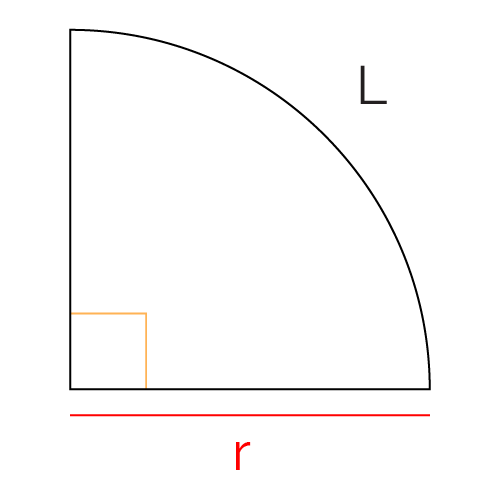# Quarter Circle Perimeter Calculator

Created by Komal Rafay
Reviewed by Anna Szczepanek, PhD
Last updated: Oct 10, 2022

The quarter circle perimeter calculator is one of the many other tools that we have made just for you shape enthusiasts. The quarter of a circle is a shape that might need your attention as there is much to know about it. Here we will be discussing its perimeter.

• What is a quarter circle?
• How to calculate the perimeter of a quarter circle? and
• The formula for the perimeter of a quarter of a circle.

## What is a perimeter of a quarter circle?

The quarter of a circle is a shape you get when you divide a circle into four equal parts. It's very similar to the sector of a circle, with the only difference being that its central angle is always 90°. This is because you divide the circle into four pieces. The complete angle of the circle is 360°, so if you divide 360 by four, it gives 90. The quarter of the circle is also called 1/4th of a circle.

Its perimeter, like any other shape, is its boundary.

## Quarter circle perimeter calculator

The quarter of a circle perimeter tool is your best bet when it comes to finding the answers with minimal effort.
All we ask from you is the radius of the circle's quarter, which in this case can also be its base. And we will give you the perimeter and, as a bonus, also the arc length.

So, maybe you are practicing your baking skills, as we all do, at some point in our lives. You decided to make a quarter circle-shaped cake instead of a whole circle (which would look so cool by the way). Now you want to cover the border,which is the perimeter, of the cake with fondant.

Say your side of the cake, which is the radius, measures $10 \text{ cm}$.
Input 10 cm as radius in our tool and the result is, $35.71 \text{ cm}$ perimeter.

Now you wrap your cake in exact measurement of fondant.

## Formula for quarter circle perimeter

The formula for a quarter of a circle perimeter is:

$P = L + 2 \times r$

where:

• $P$ - The perimeter of the quarter circle;
• $L$ - The arc length of the quarter circle; and
• $r$ - The radius of the quarter circle.

As you can see the perimeter formula depends on the arc length formula which is:

$L = \pi \times \frac{r}{2}$

Now let's put it to words and understand how to calculate the perimeter of a quarter circle.

As you can see the formulae are fairly simple to. We know the central angle, hence the only measurement needed is the radius. π times the radius divided by 2 is the arc length.

The next step is to substitute the arc length in to the perimeter formula. Arc length added to 2 times the radius is the perimeter.

## A collection of circle sector tools

The perimeter of the quarter circle calculator is only the tip of the iceberg. Take a look at some other excellent Omni tools that circle around the concept of the circle's sector!

## FAQ

### How can I calculate the perimeter of a quarter circle?

To calculate the quarter of a circle, use the formula:

P = L + 2 × r
where:

• P - Perimeter of the quarter circle;
• L - Arc length of the quarter circle; and
• r - Radius of the quarter circle.

It is really simple to use. All you have to do is:

1. Multiply the radius by 2;
2. Add it to the arc length; and
3. The result is the perimeter.

One thing to remember is that the central angle of a quarter circle is always 90° (or π).

### If the radius is 8 cm, what is the perimeter of a quarter circle?

The perimeter of a quarter of a circle is 28.57 cm, when the radius is 8 cm.

This is calculated by using the formula of the perimeter of a quarter circle:
Perimeter = Arc length + 2 × Radius

Substituting the values for arc length and radius:
Perimeter = (π × 8/2) + (2 × 8)
Perimeter = 28.57 cm

Note that the arc length formula is:
L = π × r/2

### Is quarter circle the same as sector of a circle?

The quarter of a circle can be considered the sector of a circle because they both are a segment of a circle. The distinction between the two of them is that the quarter of a circle has a central angle of 90°, whereas the sector can have any measure of the central angle.

So, in other words, every quarter circle is a sector, but not every sector is a quarter circle.

Komal Rafay
inQuarter arc (L)
in
Perimeter
in
People also viewed…

### Irregular trapezoid area

Use our irregular trapezoid area calculator to find the area of any trapezoid!

### Long subtraction

This long subtraction calculator helps you solve any long subtraction problem for any two whole numbers as well as for numbers with decimal places.

### Significant figures

The significant figures calculator performs operations on sig figs and shows you a step-by-step solution!

### Steps to calories

Steps to calories calculator helps you to estimate the total amount to calories burned while walking.